# CSCI 171 Presentation 7 Recursion What is recursion

• Slides: 23CSCI 171 Presentation 7 Recursion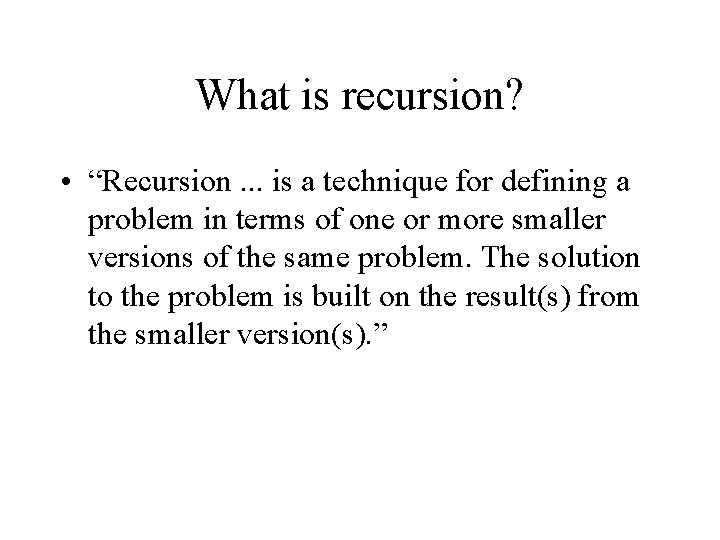What is recursion? • “Recursion. . . is a technique for defining a problem in terms of one or more smaller versions of the same problem. The solution to the problem is built on the result(s) from the smaller version(s). ”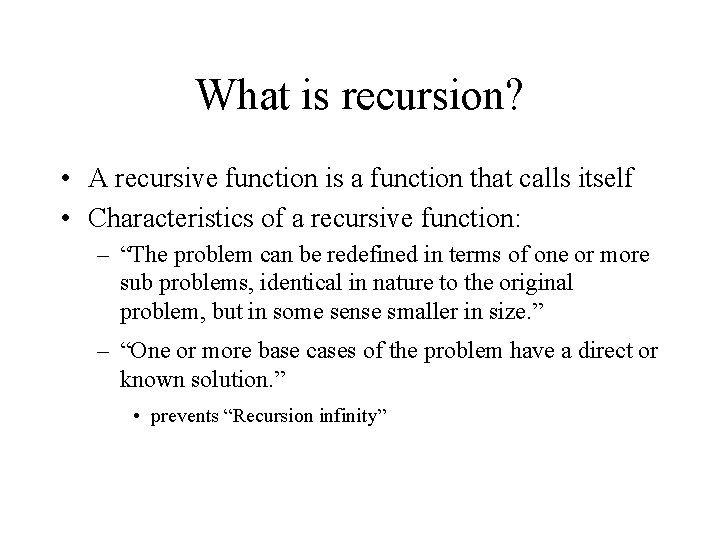What is recursion? • A recursive function is a function that calls itself • Characteristics of a recursive function: – “The problem can be redefined in terms of one or more sub problems, identical in nature to the original problem, but in some sense smaller in size. ” – “One or more base cases of the problem have a direct or known solution. ” • prevents “Recursion infinity”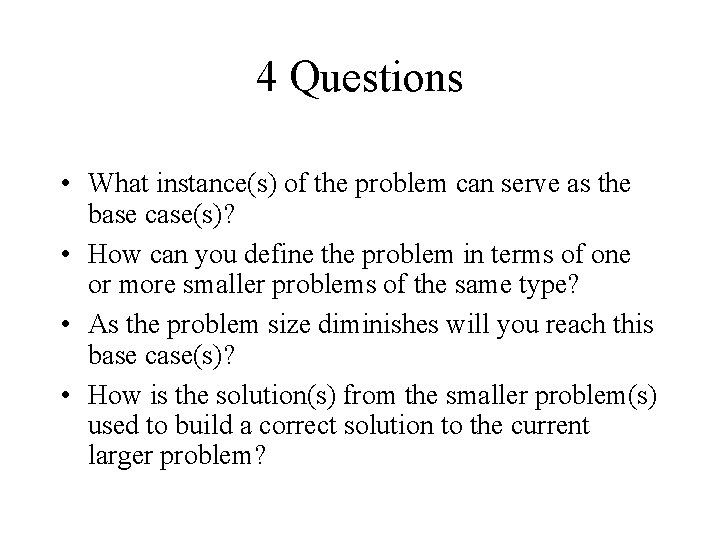4 Questions • What instance(s) of the problem can serve as the base case(s)? • How can you define the problem in terms of one or more smaller problems of the same type? • As the problem size diminishes will you reach this base case(s)? • How is the solution(s) from the smaller problem(s) used to build a correct solution to the current larger problem?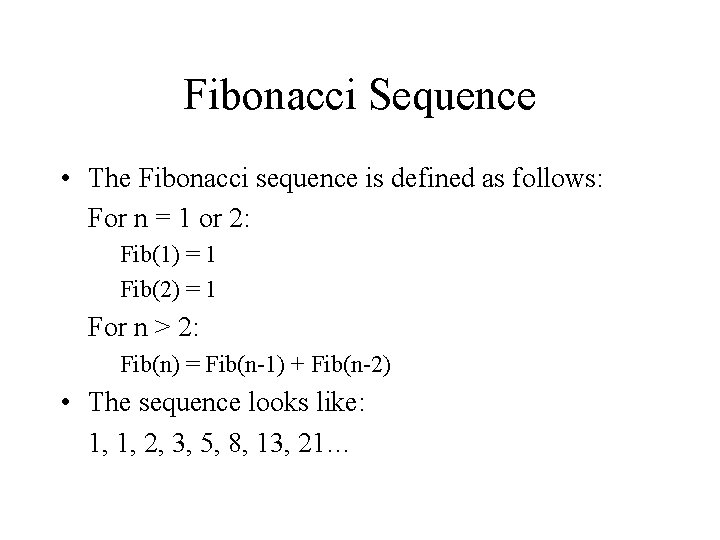Fibonacci Sequence • The Fibonacci sequence is defined as follows: For n = 1 or 2: Fib(1) = 1 Fib(2) = 1 For n > 2: Fib(n) = Fib(n-1) + Fib(n-2) • The sequence looks like: 1, 1, 2, 3, 5, 8, 13, 21…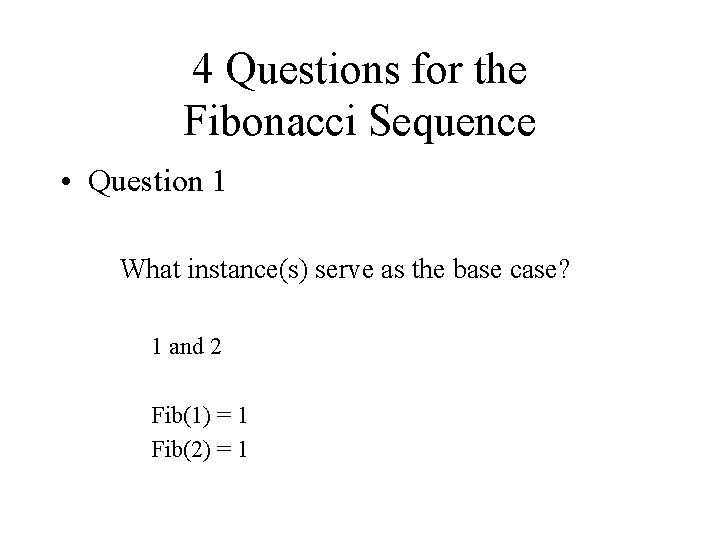4 Questions for the Fibonacci Sequence • Question 1 What instance(s) serve as the base case? 1 and 2 Fib(1) = 1 Fib(2) = 1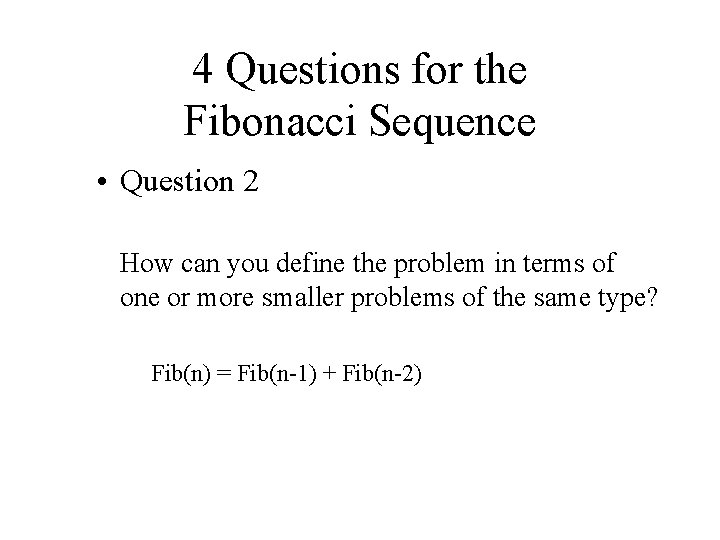4 Questions for the Fibonacci Sequence • Question 2 How can you define the problem in terms of one or more smaller problems of the same type? Fib(n) = Fib(n-1) + Fib(n-2)4 Questions for the Fibonacci Sequence • Question 3 As the problem size diminishes will you reach this base case(s)? Yes, each time you are decrementing, so you will eventually reach the base case of 1 or 2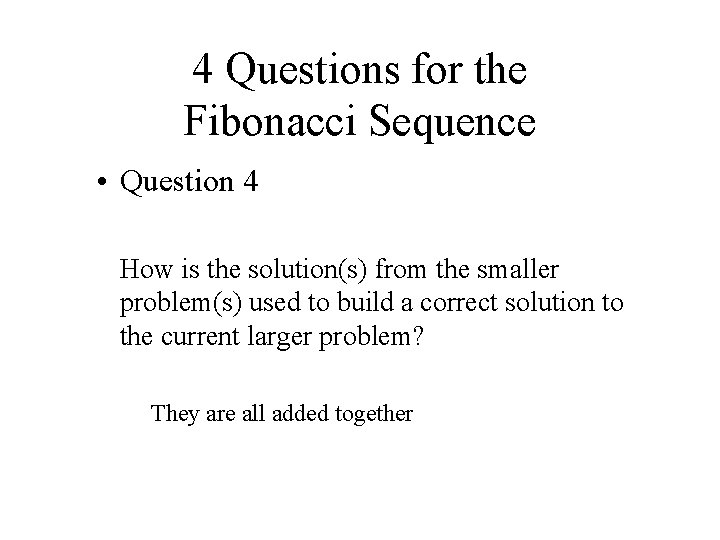4 Questions for the Fibonacci Sequence • Question 4 How is the solution(s) from the smaller problem(s) used to build a correct solution to the current larger problem? They are all added together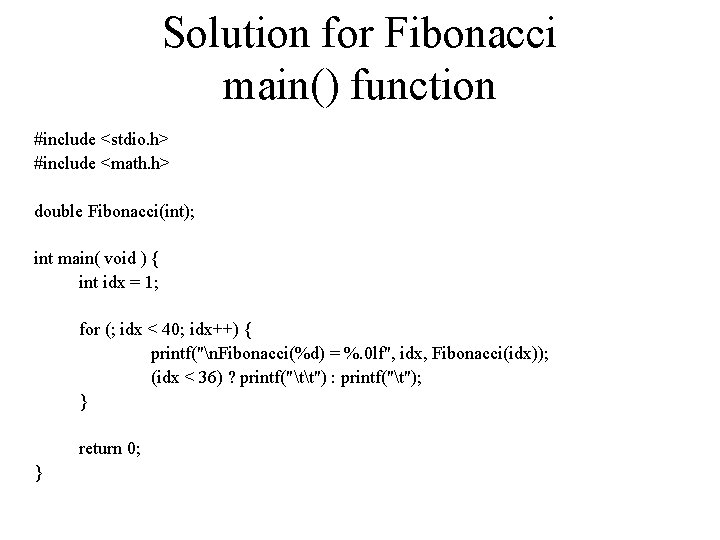Solution for Fibonacci main() function #include <stdio. h> #include <math. h> double Fibonacci(int); int main( void ) { int idx = 1; for (; idx < 40; idx++) { printf("n. Fibonacci(%d) = %. 0 lf", idx, Fibonacci(idx)); (idx < 36) ? printf("tt") : printf("t"); } return 0; }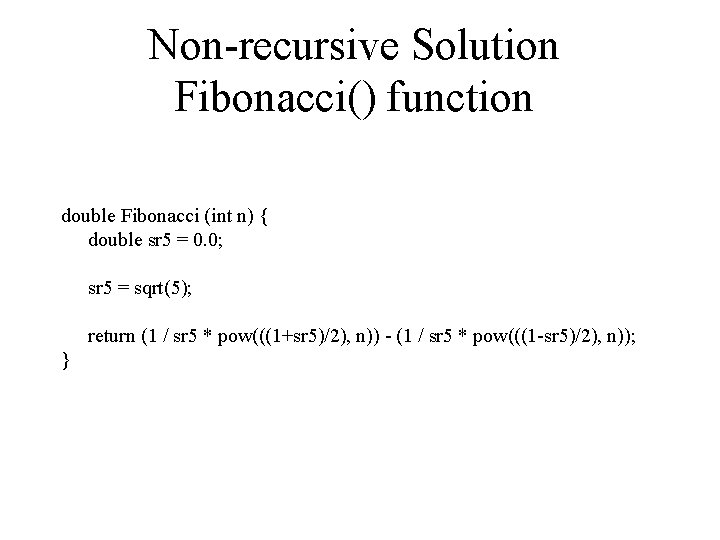Non-recursive Solution Fibonacci() function double Fibonacci (int n) { double sr 5 = 0. 0; sr 5 = sqrt(5); return (1 / sr 5 * pow(((1+sr 5)/2), n)) - (1 / sr 5 * pow(((1 -sr 5)/2), n)); }Recursive Solution Fibonacci() function double Fibonacci(int z) { if ((z == 1) || (z == 2)) return 1; else return (Fibonacci(z-1) + Fibonacci(z-2)); }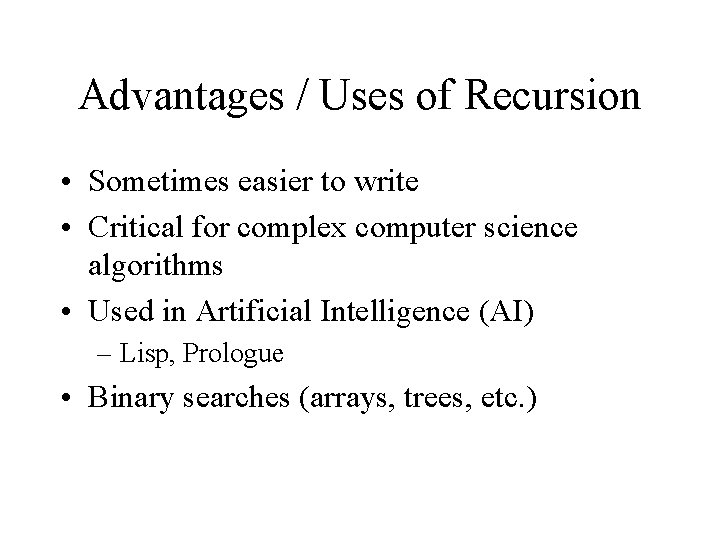Advantages / Uses of Recursion • Sometimes easier to write • Critical for complex computer science algorithms • Used in Artificial Intelligence (AI) – Lisp, Prologue • Binary searches (arrays, trees, etc. )Disadvantages of Recursion • Sometimes harder to write • Code is generally inefficient • Some languages do not support recursion – COBOL, FORTRANSample Program 9. 1. 1 #include <stdio. h> #include <math. h> double Fibonacci(int); int counter = 0; int main( void ) { int idx = 1; for (; idx < 40; idx++) { printf("n. Fibonacci(%d) = %. 0 lf", idx, Fibonacci(idx)); (idx < 36) ? printf("tt") : printf("t"); printf("The function was called %d time(s). ", counter); counter = 0; } return 0; }Sample Program 9. 1. 2 double Fibonacci(int z) { counter ++; if ((z == 1) || (z == 2)) return 1; else return (Fibonacci(z-1) + Fibonacci(z-2)); }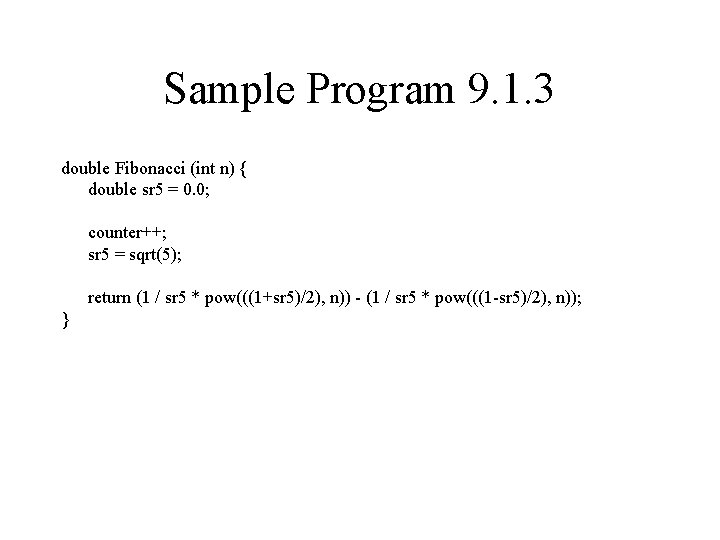Sample Program 9. 1. 3 double Fibonacci (int n) { double sr 5 = 0. 0; counter++; sr 5 = sqrt(5); return (1 / sr 5 * pow(((1+sr 5)/2), n)) - (1 / sr 5 * pow(((1 -sr 5)/2), n)); }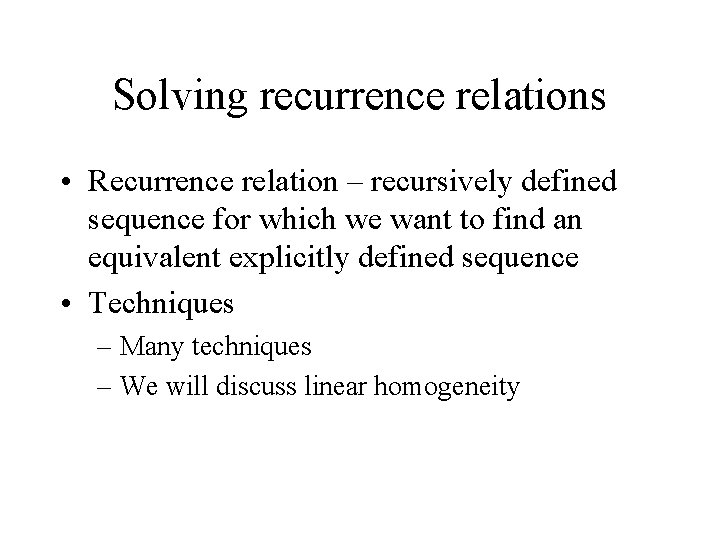Solving recurrence relations • Recurrence relation – recursively defined sequence for which we want to find an equivalent explicitly defined sequence • Techniques – Many techniques – We will discuss linear homogeneity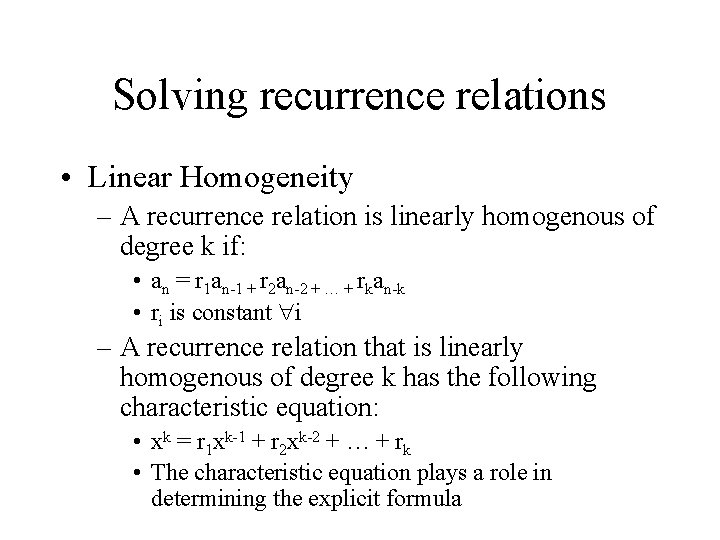Solving recurrence relations • Linear Homogeneity – A recurrence relation is linearly homogenous of degree k if: • an = r 1 an-1 + r 2 an-2 + … + rkan-k • ri is constant i – A recurrence relation that is linearly homogenous of degree k has the following characteristic equation: • xk = r 1 xk-1 + r 2 xk-2 + … + rk • The characteristic equation plays a role in determining the explicit formula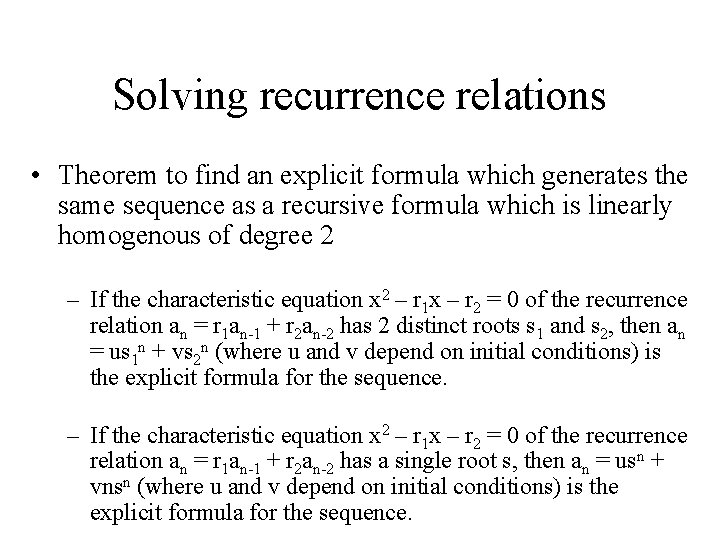Solving recurrence relations • Theorem to find an explicit formula which generates the same sequence as a recursive formula which is linearly homogenous of degree 2 – If the characteristic equation x 2 – r 1 x – r 2 = 0 of the recurrence relation an = r 1 an-1 + r 2 an-2 has 2 distinct roots s 1 and s 2, then an = us 1 n + vs 2 n (where u and v depend on initial conditions) is the explicit formula for the sequence. – If the characteristic equation x 2 – r 1 x – r 2 = 0 of the recurrence relation an = r 1 an-1 + r 2 an-2 has a single root s, then an = usn + vnsn (where u and v depend on initial conditions) is the explicit formula for the sequence.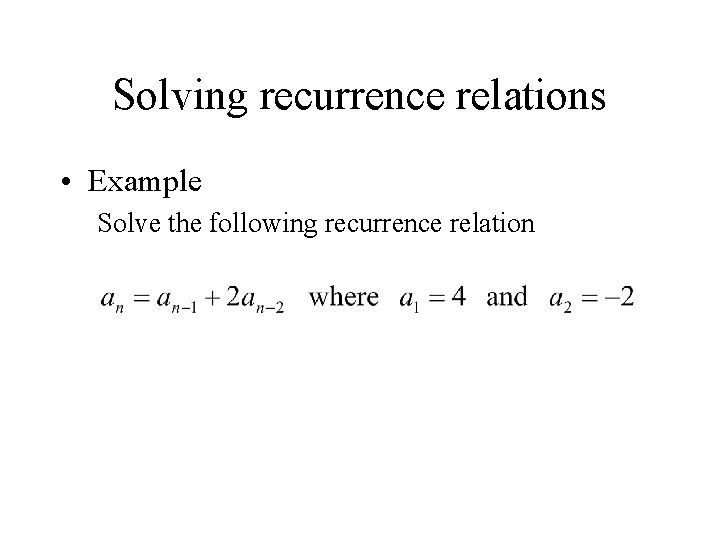Solving recurrence relations • Example Solve the following recurrence relation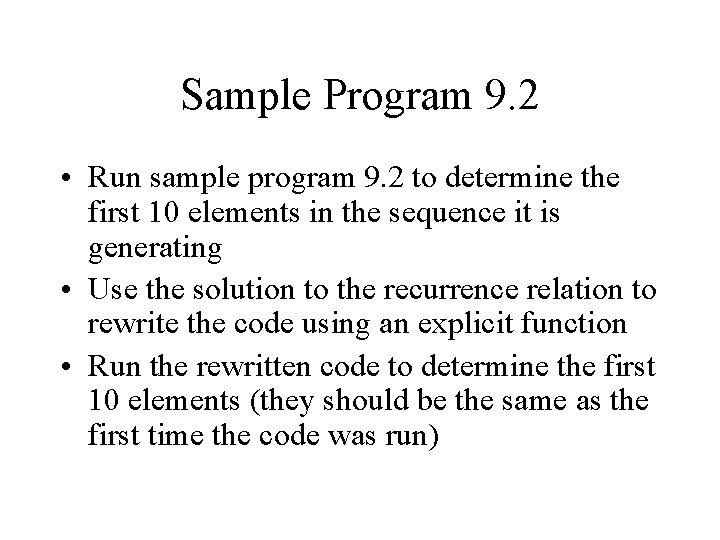Sample Program 9. 2 • Run sample program 9. 2 to determine the first 10 elements in the sequence it is generating • Use the solution to the recurrence relation to rewrite the code using an explicit function • Run the rewritten code to determine the first 10 elements (they should be the same as the first time the code was run)Recursion “Typically, solutions to problems use either iteration (looping), or recursion. In general a recursive solution is less efficient than an iterative one in terms of computer time. However in many instances, recursion provides a very natural, simple solution to a problem of great complexity. “